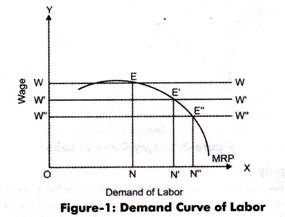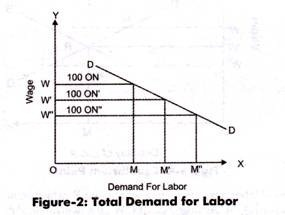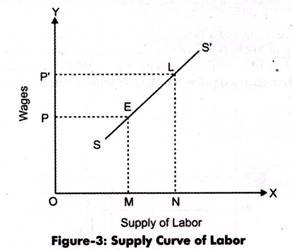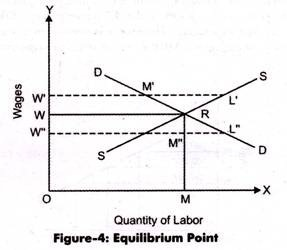# Factor of Production: Demand and Supply

Modern economists rejected the marginal productivity theory mainly because of two reasons.

Firstly, according to modern economists, the marginal productivity theory does not take into account the supply side of a factor of production.

Secondly, the marginal productivity theory is concerned only with the units of factors of production, not with the determination of prices of factors. According to modern economists, as the prices of products are determined by the interaction of two forces, demand and supply in the market.

Similarly, in perfect competition, the prices of factors of production are also determined by matching the demand and supply in the factor market. Therefore, we will discuss the two aspects of a factor of production, namely demand and supply, in the factor market.

#### Demand for a Factor of Production:

The demand for factors is a derived demand. This is because the demand for a factor of production (input) is derived from the demand of output. If the demand of output is high, then the demand for input or factor of production would also be high and vice versa.

According to the modern theory, the demand for a factor of production depends on two parameters, which are explained as follows:

i. Magnitude of demand for a factor:

Involves three conditions, which are as follows:

a. Condition 1:

Implies that there would be high demand for a factor of production if it is highly important in the production process.

b. Condition 2:

Implies that there would be high demand for a factor of production if the demand for output or final product is high.

c. Condition 3:

Implies that there would be low demand for a factor of production if it has close substitutes.

ii. Elasticity of demand for a factor:

Refers to the responsiveness of demand for a factor with change in its price.

The elasticity of demand for a factor also depends on three conditions, which are as follows:

a. Condition 1:

Implies that if the price of a factor is very low with respect to the total cost, then the demand for that factor will be inelastic and vice versa.

b. Condition 2:

Implies that if the demand for the product for which the factor of production is used is elastic, then the demand for the factor used would also be elastic.

c. Condition 3:

Implies that if the factor of production has easy availability of substitutes in the market, then its demand would be highly elastic. Now let us discuss the individual demand curve of a factor of production.The demand curve of a factor of production is determined with the help of MRP. Here, we take the example of labor and wages to draw the individual demand curve. The demand for labor is determined by an employer with the help of MRP and prevailing wage rates. In case, the wage rate is low, then the labor employed would be higher and vice versa.

Figure-1 shows the demand curve of labor:In Figure-1, when the wage rate is OW and the demand for labor is ON, then the equilibrium is attained at point E. Similarly, at point E’, the wage rate is OW and demand for labor is ON’ and at E” the wage rate is OW” with demand for labor ON”. MRP represents the demand curve for an individual organization. However, it is required to determine the market demand for labor.

The market demand curve can be derived by adding up the MRP curves of different organizations in an industry-, which is shown in Figure-2:In Figure-2, DD curve shows the market demand for labor in an industry. It is assumed that the number of organizations in an industry is 100. In Figure-2, when the wage rate for an individual organization is OW, then the demand for labor was ON.

However, in Figure-2, when the wage rate for an industry is OW, then the demand for labor is OM = 100 ON, which is the demand for labor for 100 organizations in the industry). Similarly, when the wage rate of industry is OW then demand of labor is OM’ (=100 ON’) and at OW” it is OM” (=100 ON”). The downward slope of demand curve DD represents that increase in labor would result in the decrease of marginal productivity.

#### Supply of a Factor of Production:

After discussing the demand for a factor of production, it is important to understand its supply, so that the price of the factor can be determined. Determining the supply of factors of production is a complex task as each type of factor creates a problem. For example the quantity of land is fixed, thus its supply cannot be increased or decreased with change in its prices.

Similarly, if we analyze the total supply of labor in a country, it depends on a number of factors, such as size and composition of population, efficiency of labor, geographical distribution, expected wages, and educational qualifications.

In such a case, the total supply of labor is fixed; however, it can be increased by increasing the working hours of labor employed. Moreover, the supply of capital also depends on factors, such as rate of interest, saving capacity of individuals, and their willingness to save. Therefore, in short, it can be said that the supply of a factor is also a function of price.

If the price of a factor of production is higher, then its supply would also be higher while others factors are constant and vice versa. Therefore, the slope of the supply curve of a factor of production is upward to right. Here, again we take the example of labor and wages to draw the supply curve.

Figure-3 shows the supply curve of labor:#### Interaction of Demand and Supply:

According to the modem theory, the price of a factor of production is determined at a point where the demand and supply curves of the factor intersect each other. This point is known as equilibrium point, where the demand of a factor is equal to its supply.

Figure- 4 shows the equilibrium point where the price of a factor of production is determined:In Figure-4, demand and supply curves of labor intersect each other at point R, which is the equilibrium point. At point R, the wage rate is OW (=MR) and demand for labor is OM. At wage rate of OW, the demand for labor is W’M’, which is less than its supply. This implies that there is surplus of labor in the market.

For overcoming the situation, the wage rate falls down to OW (where the equilibrium is attained). Similarly, if the wage rate is OW”, then the demand for labor is W”L”, which is more than its supply. This implies that there is a shortage of labor in the market. In such a case, the wage rate increases to OW. Finally, we reach the equilibrium point at which the demand and supply of labor is equal. At this point, the wage rate for labor is determined.

The modern theory of factor pricing was an attempt to make improvement in marginal productivity theory.

However, the modern theory is criticized due to its weak assumptions, which are as follows:

i. Assumes only perfect competition in both the product market as well as the factor market. However, in real situations, both of these markets face imperfect competition.

ii. Assumes that there is homogeneity in all the factors of production, which is not true in real market scenario.

iii. Assumes that all factors of production have close substitutes, which is not always possible.